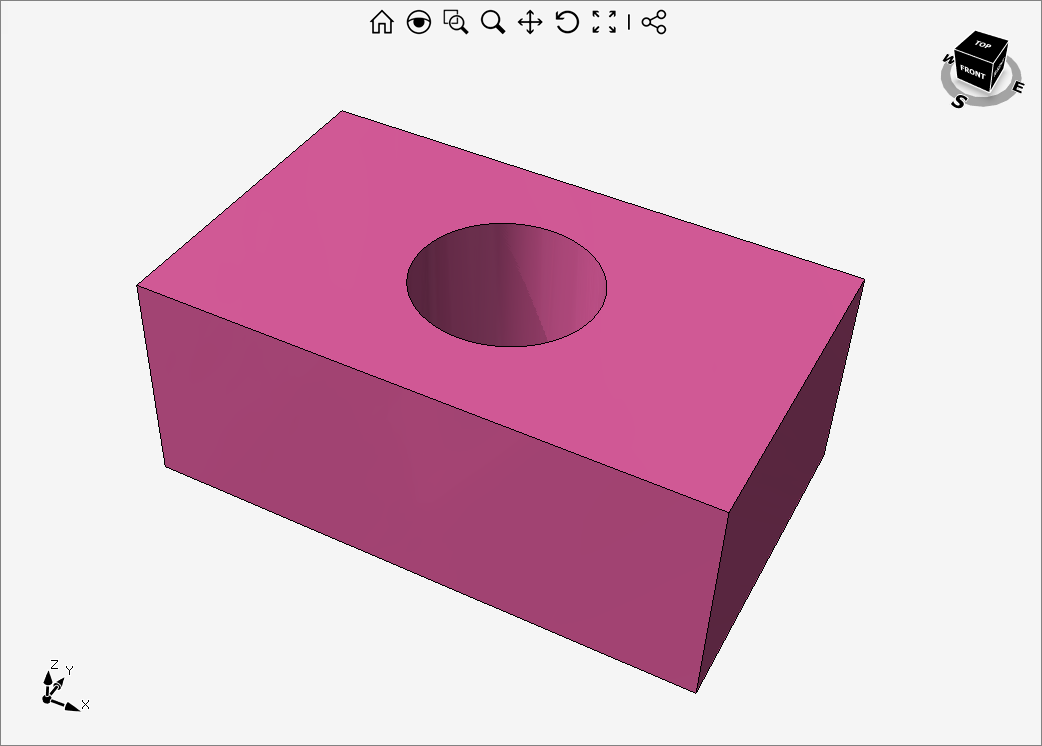# Changing hole diameter in a BRep objectIn the following code, we first create a BRep object with a hole, then we change the diameter of a hole with world axis-alignment by editing BRep elements: faces/edges/vertices.

Note that this code will work only for radius values that do not intersect/touch the contours of the others faces.

``````devDept.Eyeshot.Entities.Region reg = devDept.Eyeshot.Entities.Region.CreateRectangle(50, 30);

GCircle c1 = new GCircle(25, 15, 0, radius);

c1.Reverse();

const double amount = 20;

Brep brep = reg.ExtrudeAsBrep(amount);

// changes the cylindrical face radius
foreach (var face in brep.Faces)
{
if (face.Surface is TabulatedSurf ts && ts.Directrix is GCircle cr)
{
}
}

// resizes circular edges and moves the seam curve (line)
foreach (var edge in brep.Edges)
{
if (edge.Curve is GArc cr)
{
}
else if (edge.Curve is GLine ln && edge.StartPointIndex == 8)
{
}
}

// moves the two vertices on the cylindrical face's seam
for (int i = 0; i < brep.Vertices.Length; i++)
{
if (i == 8 || i == 9)
{
var vertex = brep.Vertices[i];

}
}

brep.RegenMode = regenType.RegenAndCompile;

design1.Entities.Regen();``````

Below, a sample code for changing the diameter of a particular selected face (with any orientation), by tracing all the needed data directly from the Face loops structure.

``````devDept.Eyeshot.Entities.Region reg = devDept.Eyeshot.Entities.Region.CreateRectangle(50, 30);

GCircle c1 = new GCircle(25, 15, 0, radius);

c1.Reverse();

const double amount = 20;

Brep brep = reg.ExtrudeAsBrep(amount);

int selectedFaceIndex = 4;

GBrep.Face selectedFace = brep.Faces[selectedFaceIndex];

bool cylTabulated = (selectedFace.Surface is TabulatedSurf ts && ts.Directrix is GCircle);
bool cylinder = selectedFace.Surface is CylindricalSurf;
if (cylTabulated || cylinder)
{
Vector3D axisX;
Segment3D axis;
// changes the cylindrical face radius and get the axes data
if (cylTabulated)
{
GCircle directrix = (GCircle)((TabulatedSurf)selectedFace.Surface).Directrix;
axisX = directrix.Plane.AxisX;
axis = new Segment3D(directrix.Plane.Origin, directrix.Plane.Origin + directrix.Plane.AxisZ);
}
else
{
CylindricalSurf cs = (CylindricalSurf)selectedFace.Surface;
selectedFace.Surface = new CylindricalSurf(cs.Plane.Origin, cs.Plane.AxisZ, cs.Plane.AxisX, newRadius, cs.Index);
axisX = cs.Plane.AxisX;
axis = new Segment3D(cs.Plane.Origin, cs.Plane.Origin + cs.Plane.AxisZ);
}

foreach (GBrep.Loop loop in selectedFace.Loops)
{
foreach (GBrep.OrientedEdge orientedEdge in loop.Segments)
{
GBrep.Edge edge = brep.Edges[orientedEdge.CurveIndex];

if (edge.Curve is GArc arc)
{
// resizes circular edges

// moves the two vertices on the cylindrical face's seam
brep.Vertices[edge.StartPointIndex] = arc.StartPoint;
brep.Vertices[edge.EndPointIndex] = arc.EndPoint;
}
else if (edge.Curve is GLine ln)
{
// moves the seam curve(line)
Point3D startOnAxis = axis.PointAt(axis.Project(ln.StartPoint));
Point3D endOnAxis = axis.PointAt(axis.Project(ln.EndPoint));

ln.StartPoint = startOnAxis + axisX * newRadius;
ln.EndPoint = endOnAxis + axisX * newRadius;
}
}
}
}

brep.RegenMode = regenType.RegenAndCompile;

design1.Entities.Regen();``````
1 out of 1 found this helpful
Have more questions? Submit a request

• Nice example!
Would be great, if the indices weren't used directly, and there were code that actually makes the connection between the TabulatedSurf directrix and the Brep elements, that need to be updated, afterwards...

That would give people a better understanding of how the Eyeshot Brep works :)

• Hello Martin,

Are you referring to this statement?

` if (i == 8 || i == 9)`
• Hi Alberto,
yes, and the

``edge.StartPointIndex == 8``

aswell...

• You can find the geometry in the way you like, if you have a selected face you can find its loops and edges using Face.Loops property. In this specific case, you can try using Edge.GetStartPointIndex() or GetEndPointIndex().

• Exactly, that's what I'm talking about :)

• Hi Martin,

We have updated the source code for the case on which the only information needed is the index of the face you want to change.